Détails du magazine
Format
Magazine
eISSN
1891-5469
Première parution
01 Jan 2010
Périodicité
1 fois par an
Langues
Anglais
Accès libre

# Positive phase error from parallel conductance in tetrapolar bio-impedance measurements and its compensation

###### Reçu: 05 Nov 2010
Détails du magazine
Format
Magazine
eISSN
1891-5469
Première parution
01 Jan 2010
Périodicité
1 fois par an
Langues
Anglais
Introduction

Accuracy and precision are paramount in bioimpedance measurements (1). Electrode polarisation effects arise at electrode surfaces due to the transition between electronic and ionic current flow. In the case of a two-electrode impedance measurement, these effects are superimposed on the actual impedance of the material being investigated. Schwan proposed the tetrapolar approach as a means of removing electrode polarisation effects (2). However, this approach is not devoid of measurement error. Residual electrode polarisation effects are one source of error (3; 4). Others identified are common mode signals and alternative current paths (5; 6). The non-uniform sensitivity of the measurement set-up also plays a key role in determining the measured impedance since it is now the transfer impedance between the current carrying (CC) and pick-up (PU) electrodes that is being measured (7; 8).

In this work we first characterise, through simulation, the error that results from the existence of a parallel conductive pathway in the measurement set-up. Next, we explore the possibility that experimental tetrapolar measurement errors can be represented by such a parallel conductance. Spectral measurements have been made using a fluid filled well chamber and these give a good fit to spectra derived from equivalent electrical circuit modelling incorporating a parallel conductance. The validity of the model is assessed through 3D finite element modelling (FEM) of the measurement device.

In biological investigations positive phase characteristics are unlikely to be a genuine reflection of the underlying electrical properties of the sample. The typical Cole-Cole model of cells (9) depicts a biological sample as having two resistive components in parallel, one corresponding to the extracellular space and another corresponding to the intracellular space. In series with the intracellular resistance is a capacitive component to model the barrier and charge-storing properties of the cell membrane. Cellular samples therefore display a characteristic !-dispersion from high to low impedance with increasing frequency (10; 11). Here we show experimental measurements from cell cultures placed in the well chamber in which the !-dispersion is occluded by artefact and use the same equivalent electrical circuit model to extract the underlying !-dispersions more accurately.

Parallel Conductive Pathway

The basic set-up for a tetrapolar, or four-electrode, impedance measurement consists of two current carrying electrodes used to inject alternating current, I, into the sample and two additional electrodes to pick-up the voltage that is produced across the sample, VPU. The measured impedance of the sample, ZM, is then calculated as the complex ratio of the pick-up voltage to the injected current: ZM = VPU / I.

If a parallel conductive pathway exists the measuring circuit is modified as in figure 1. In this case the current injected into the sample is reduced to the new value iS and there will be a concomitant drop in the voltage measured across the sample. The discrepancy between the actual sample impedance and the measured impedance results from the fact that the full injected current is used to calculate the measured impedance. The existence of a parallel current pathway means that the current flowing through the sample is over-estimated such that the measured impedance is less than the true sample impedance. It will be shown that this artefact has the appearance of an inductance having increasing impedance magnitude with frequency and positive phase at low to intermediate frequencies.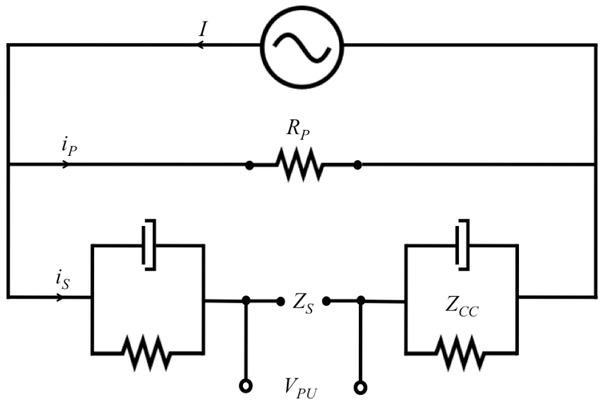A parallel conductive pathway with resistance, RP, in the measurement circuit reduces the current reaching the sample, iS, by an amount, iP, corresponding to the current flowing through this extraneous pathway. The full injected current, I, is used to calculate the sample resistance leading to measurement error.
Materials and methods
Simulations

Circuit analysis of the set-up depicted in figure 1 shows that, in the presence of a parallel conductive pathway, the current reaching a sample with impedance ZS is reduced by a factor given by the ratio of the total impedance of the circuit, ZTOT, to the impedance of the pathway containing the sample:

$is=ZTOTZCC+ZSI$$${{i}_{s}}=\frac{{{Z}_{TOT}}}{\left( {{Z}_{CC}}+{{Z}_{S}} \right)}I$$

Here ZCC is the total impedance of the current carrying electrodes. The voltage measured by the pick-up electrodes is given by:

$VPU=iSZS$$${{\mathbf{V}}_{PU}}={{i}_{S}}{{Z}_{S}}$$

These expressions can be combined to give an expression for the measured impedance:

$ZM=VPUI=ZTOTZCC+ZSZS$$${{Z}_{M}}=\frac{{{\text{V}}_{PU}}}{I}=\frac{{{Z}_{TOT}}}{\left( {{Z}_{CC}}+{{Z}_{S}} \right)}{{Z}_{S}}$$

Simulations were performed in MatLab (The Mathworks, UK) to examine the role played by the various components present in this expression and to characterise the distortion to the impedance spectra that results.

Measurement Rig

Experimental data was acquired using an EndOhm-12 device (World Precision Instruments, UK) designed for trans-epithelial electrical resistance measurements of cell samples grown on transwell membranes. Figure 2 shows a schematic of the measurement rig containing solution but no transwell membrane. The corresponding cross-sectional geometry, axially symmetric about r = 0, is also shown. The pick-up (PU) electrodes are symmetrically apposed Ag/AgCl sintered pellets 2mm in diameter. One is centred in the base of the well chamber. The second is centred in a pillar in the lid of the device, which can be inserted into the well chamber. The current-carrying (CC) electrodes are circular strip sterling silver electrodes 4.2mm wide in the base and 1mm tall in the pillar. Upon fabrication, the CC electrodes are coated with a bleach-generated chloride layer (Clorox, California, USA).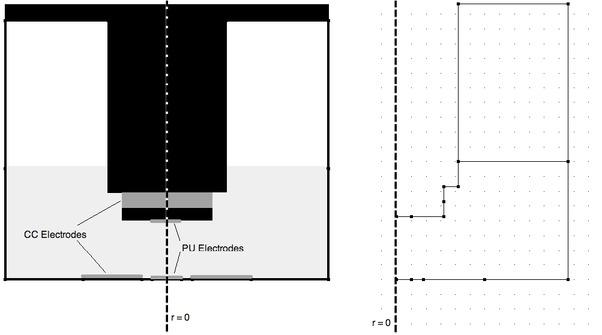Schematic cross-section of the EndOhm-12 device (left) used to acquire impedance spectra and the corresponding 2D model geometry (right) that is symmetric about the r = 0 axis allowing parameters to be evaluated over the full 3D volume. The nodal points demark the extent of geometry elements.
Impedance Measurements

The EndOhm device is designed for single frequency resistance measurements but was interfaced with a freshly calibrated Solartron 1260 impedance analyser (Advanced Measurement Technology, UK) for spectral analysis. Electrical impedance spectroscopy measurements were made at logarithmically spaced frequencies in the range from 100Hz to 1MHz with ten points per decade. A 1V signal was applied. The current carrying and pick-up circuitry were connected by a common ground.

Artefact seen in the spectra was confirmed as being independent of the Solartron analyzer by using a separate current source and monitoring the voltage on the pick up electrodes with voltage probes and an oscilloscope.

The chloride layer initially coating the CC electrodes suffers thinning over time, which does not recondition fully upon re-chloriding. To examine the effect of contact impedance on the acquired spectrum, spectra with and without (one month later) the chloride layer being present on the CC electrodes were compared. The data were acquired after filling the main well chamber of the device with 3ml of phosphate buffered saline (PBS) (Sigma, UK); inserting a blank transwell membrane (Corning Inc., NY, USA) and adding 0.5ml PBS to the transwell centre.

To validate the fitting procedure the EndOhm device was filled with 5ml of commercially available known-conductivity calibration solutions (Hanna Instruments, UK). No transwell membranes were used in these cases. Conductivities ranging from 0.14S/m to 1.28S/m were used to correspond with the physiological range. The temperature was recorded and the corresponding conductivity was determined from the solution’s technical sheet. The lid was seated on the device allowing full submergence of the upper electrodes.

Finite Element Modelling

The finite element model (FEM) of the EndOhm device was constructed in Comsol Multiphysics (Comsol AB, Sweden) using the AC/DC module under quasi-static conditions. The cylindrical geometry of the device was simplified to a 2D axially symmetric model (figure 2, right) The upper electrode being used for current injection was set to the port boundary condition with a fixed current input, while the lower CC electrode was set to ground. The pick-up electrodes were set to the floating potential boundary condition. To match the experimental conditions used for the calibration solutions, the well chamber was modelled as being filled with 5ml of solution and with the upper pillar separated from the base of the chamber by 4.3mm. The sub-domain corresponding to the solution was set to the appropriate temperature-specific conductivity. No permittivity information was available from the manufacturers so the relative permittivity was set to 80, a typical value for liquids such as water and saline. The initial model consisted of 344 triangular elements. Adaptive mesh refinement was used when solving the model to maximise accuracy. The final mesh consisted of 2,734 elements.

When making tetrapolar impedance measurements, it is the transfer impedance between the CC and PU electrodes that is measured meaning that the sensitivity of the measuring device should be considered (5; 7; 8). The sensitivity, S, of the device measures the contribution of each element within the volume to the overall impedance measured and was calculated as:

$S=JCC⋅JPUICCIPU$$$S=\frac{{{J}_{CC}}\cdot {{J}_{PU}}}{{{I}_{CC}}{{I}_{PU}}}$$

Here JCC is the current density that results by using the CC electrodes for current injection and JPU is the current density produced by reversing the set-up and using the PU electrodes for current injection. I is the integral of the normal current density injected through the surface, δΩ, of the particular electrode being used for current injection. For example:

$ICC=∫∂Ω−JCC⋅n^dS$$${{I}_{CC}}=\int\limits_{\partial \Omega }{-{{J}_{CC}}\cdot \hat{n}dS}$$

Positive sensitivity means that an increase in the resistivity of the sample will result in a corresponding increase in the measured resistance. Conversely, negative sensitivity results in a decrease in the measured resistance for the same increase in resistivity. Since the sensitivity is normalised to the current level, this distribution is in fact independent of the current level used.

The geometric factor or cell constant of a measurement rig is the coefficient of proportionality between the resistivity, ρ, of a homogeneous sample and the measured transfer resistance, R. The calculated sensitivity from the FEM can be used to calculate the pseudo geometric factor of the measurement rig being modelled. This factor, g, is calculated by summing the contribution from all volume elements, dv, throughout the measurement volume V:

$RSEN=∫VJCC⋅JPUI2ρdV=gρ$$${{R}_{SEN}}=\int\limits_{\text{V}}{\frac{{{J}_{CC}}\cdot {{J}_{PU}}}{{{I}^{2}}}}\rho dV=g\rho$$

This expression was used to calculate the expected transfer resistance of each known conductivity solution.

A second measure of the expected transfer resistance was calculated from the FEM by numerically implementing the measuring procedure. First, the voltage on the upper and lower PU electrodes was calculated as:

$V=∫∂Ω2πrVdS∫∂Ω2πrdS$$$V=\frac{\int\limits_{\partial \Omega }{2\pi r\text{VdS}}}{\int\limits_{\partial \Omega }{2\pi r\text{dS}}}$$

The expected transfer resistance was calculated by dividing the voltage difference, corresponding to VPU, by the current injected through the CC electrode (as calculated above):

$RFEM=VUPPER−VLOWERICC$$${{R}_{FEM}}=\frac{{{V}_{UPPER}}-{{V}_{LOWER}}}{{{I}_{CC}}}$$
Equivalent Electrical Circuit Fitting

Fitting to the equivalent electrical circuit model of parallel conductance depicted in figure 1 was performed in MatLab using the Neldr-Mead simplex algorithm (12). The fitting was carried out with nine free parameters as follows. First, a resistance, RS, and capacitance, CS are used to represent the solution impedance:

$Zs=1Rs+jωCs−1$$${{Z}_{s}}={{\left( \frac{1}{{{R}_{s}}}+j\omega {{C}_{s}} \right)}^{-1}}$$

Here ω is the angular frequency and j = \$-1. Each of the CC electrodes is represented with a charge transfer resistance, RCT, and a constant phase element (13; 14) with double layer capacitance, Cdl, and exponent, α, to capture the inhomogeneous nature of the electrode surface:

$ZCC=1RCT+jωCdlα−1$$${{Z}_{CC}}={{\left( \frac{1}{{{R}_{CT}}}+{{\left( j\omega {{C}_{dl}} \right)}^{\alpha }} \right)}^{-1}}$$

A resistance, RP, represents the parallel conductive pathway. The total impedance incorporating the solution, the CC electrodes and the parallel pathway is calculated as:

$ZTOT=1Rp+1ZCCUPPER+ZS+ZCCLOWER−1$$${{Z}_{TOT}}={{\left( \frac{1}{{{R}_{p}}}+\frac{1}{{{Z}_{C{{C}_{UPPER}}}}+{{Z}_{S}}+{{Z}_{C{{C}_{LOWER}}}}} \right)}^{-1}}$$

Finally the fitted impedance spectrum, ZF, is:

$ZF=ZSZTOTZCCUPPER+ZS+ZCCLOWER$$${{Z}_{F}}={{Z}_{S}}\frac{{{Z}_{TOT}}}{\left( {{Z}_{C{{C}_{UPPER}}}}+{{Z}_{S}}+{{Z}_{C{{C}_{LOWER}}}} \right)}$$

Given the complex nature of the acquired data, optimisation was achieved by minimising the sum of squared differences between the measured, ZM, and fitted, ZF, data for both the real and imaginary components as per the following cost function:

$min∑NReZF−ZM2+ImZF−ZM2$$$\text{min}\left( \sum\limits_{N}{\left( \operatorname{Re}{{\left( {{Z}_{F}}-{{Z}_{M}} \right)}^{2}}+\text{Im}{{\left( {{Z}_{F}}-{{Z}_{M}} \right)}^{2}} \right)} \right)$$

Here N is the total number of spectral data points acquired. The fitting procedure is looped with the result of the fit being used as the starting point for subsequent iterations to minimise the risk of the fitting procedure stopping prematurely due to a local minimum. To ensure a physically realistic fit, only positive values were allowed for each of the fitting parameters and the alpha parameter was restricted to the range from 0.6 to 1.

Cell Layer Measurements

Impedance spectra of cell cultures were acquired using a human colorectal tumour cell line (HCT116). These were grown until confluent on collagen treated polytetrafluoroethylene (PTFE) membranes (Corning Inc., NY, USA) that promote cell attachment and spreading. The transwell insert diameter is 12mm. The insert’s membrane has a growth area of 1.12cm2 with 0.4μm pores and a nominal thickness of 30μm. It rests on the upper lip of the well chamber and extends approximately 1.6cm into the device with the membrane of the transwell positioned between the upper and lower electrodes. Cell layers of different density were grown by seeding the transwell membranes at four different levels: approximately confluent, 33%, 11% and 4% coverage. Two wells were used for each seeding level. Two blank transwells were also incubated to provide a baseline measurement under equivalent conditions. After four days growth, the cells were removed from the incubator and allowed to reach equilibrium with room temperature for a period of one hour. Each transwell was then washed twice with PBS. Care was taken to ensure that the cell layers did not lift off the transwell membrane during washing. The transwells were then inserted into the EndOhm device containing 3ml of PBS (replenished for each transwell membrane). A further 0.5ml of PBS was added to the transwell to cover the cells and create a contact between the upper and lower electrodes. With this procedure, the solution inside and outside of the transwell was approximately level. Micrographs were acquired before and after the impedance measurements using a Nikon Diaphot microscope running LUCIA G software and equipped with a DXM1200F camera. The cells were then lysed and the absorbance of the samples at 280nm was measured using a Helios Zeta spectrophotometer (Thermo Scientific, UK). The optical density was converted to cell number based on a calibration curve. The calibration curve was determined by performing linear regression on optical density measurements acquired using cell extracts for which the cell numbers had been established using conventional cell counting with a hemocytometer. Figure 3 shows an example micrograph from a well seeded at 11% confluency and the equivalent electrical circuit used to model the transwell membranes.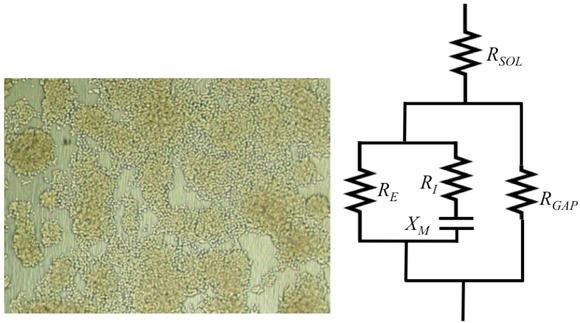Micrograph of a non-confluent transwell membrane (left) and the proposed equivalent electrical circuit (right) for the EndOhm device with the transwells in situ. RSOL represents the solution resistance above and below the transwell membrane. RGAP represents the areas of the transwell on which no cells have grown. The pathway through the cells is represented by the conventional Cole model.

From the micrograph of figure 3 it can be seen that there are areas of the transwell membrane to which no cells have adhered. In the equivalent electrical circuit of the transwell membrane these areas are collectively represented by a resistance, RGAP. As the foci merge the area of blank transwell membrane is reduced. Where cells have grown, the cell density is non-uniform. These regions are represented by the conventional cell model, in which the extracellular space is represented by a resistance denoted RE; the intracellular space is represented by a resistance denoted RI; and the capacitive nature of the cell membrane is represented by a reactance denoted XM. The solution above and below the transwell membrane is represented by the resistance, RSOL, in series with the transwell membrane layer. The sample impedance, ZS, introduced above for situations where the device is filled only with solution was replaced by this model in order to extract the electrical properties of the transwell membranes from the spectra.

As there are a large number of parameters in this model, the result is sensitive to the initial conditions. Fitting was begun with a blank transwell membrane and a gap resistance of 0# since this would be the condition to bypass the cellular compartment of the model. Each subsequent transwell was initialised with the result from the previous fit, with the exception that the gap resistance was incremented by 50# for the first seeding level to reflect the fact that the resistance of these zones will increase with the existence of cell foci. The extracellular pathway is preserved via the RE parameter of the conventional cellular model.

Results
Simulations

Figure 4 shows a simulation to contrast the appearance of artefact due to electrode polarisation effects with artefact caused by the presence of a parallel conductive pathway. Electrode polarisation effects (+) in the impedance spectrum diminish with increasing frequency as the double layer capacitance has greater bearing on the coupling to the sample. At sufficiently high frequency the measured impedance converges on the true sample impedance, simulated as 500# and zero phase in this instance (□).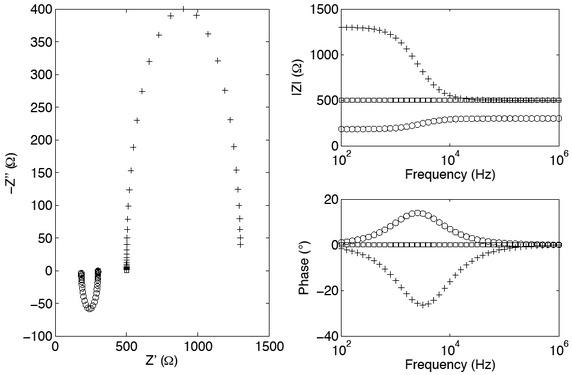In these simulations of the circuit shown in figure 1, the true sample impedance is purely resistive with no phase component (□). Artefact caused by electrode polarisation effects (+) is characterised by negative phase and decreasing impedance magnitude with frequency. In contrast, artefact due to a parallel conductive pathway (o) is characterised by positive phase and increasing impedance magnitude with frequency. The parallel conductance is 750#. Each electrode is characterised by a charge transfer resistance of 800# and a double-layer capacitance of 0.1μF.

Conversely, in the case of a parallel conductance (o), the measured impedance does not converge on the true sample impedance. The measured impedance is inversely dependent on the polarisation impedance of the CC electrodes introducing a frequency dependence, even for the case of a purely conductive parallel path and a purely conductive sample. As the frequency increases, the electrode impedance will decrease and the current flowing through the sample will increase. This results in the measured impedance increasing in magnitude and having positive phase. As the frequency increases further, the current flowing in the sample will approach the actual injected current level and the measurement error will reduce. The measured impedance would only converge on the true sample impedance when the resistance of the parallel pathway increases sufficiently to satisfy the open circuit condition, i.e. infinite impedance.

Further simulations in which a parallel conductance is present are shown in figure 5. These simulations examine the effect of the CC electrode contact impedance and the resistance of the parallel pathway on the impedance spectrum. For each of the scenarios presented, the true sample impedance (again 500# and no phase) is never measured accurately.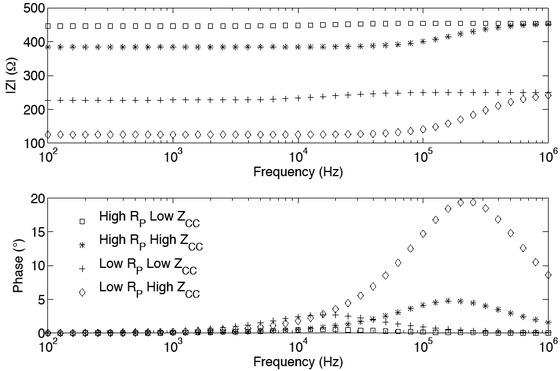The double layer capacitance, charge transfer resistance and parallel resistance are set to 1nF, 1kΩ and 5kΩ respectively for the “high” condition and to 100nF, 100# and 500# respectively for the “low” condition. The true sample impedance is purely resistive at 500#.

For the cases of CC electrodes with high contact impedance, the measurement error is immediately apparent because of the increase in impedance magnitude and peaking phase profile above 100kHz. The error is accentuated when the resistance of the parallel pathway is low. In this case the variability is over 80% and the mean error across the spectrum is over 70%. When the CC electrodes have low contact impedance, the phase profile is much flatter, being less than three degrees across the full spectrum in these examples. The magnitude of the measured impedance spectrum is correspondingly more uniform and the variability drops to below 10%. However, the measurement error is still considerable and reaches over 50% when the parallel pathway is of low resistance. The variability and mean error for each case is quantified in table 1.

The variability of the impedance magnitude is calculated with the range expressed as a percentage of the mean. The mean error of the magnitude is with respect to the true sample impedance.

CaseVariability (%)Mean Error (%)
High ZCC High RP17.0020.35
High ZCC Low RP80.5371.14
Low ZCC High RP1.809.98
Low ZCC Low RP9.5752.53
Experimental Data

Figure 6 shows typical data acquired from the EndOhm device with a transwell membrane in situ. In the time that lapsed between measurements the chloride coating was stripped from the lower CC electrode. In keeping with simulated data, the variability in the spectrum increased from 6.84% for the chlorided case to 29.86% for the dechlorided case. The solid lines show the result of fitting this data to the model of parallel conductance. The fitting results show a dramatic rise in contact impedance for one of the electrodes. This is consistent with the fact that the chloride layer has been stripped from the lower CC electrode.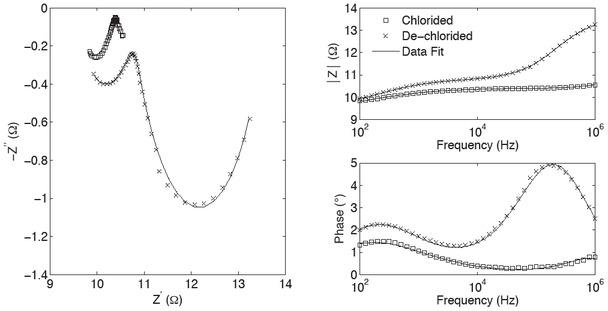Blank transwell membrane data with and without a chloride layer on the surface of the lower CC electrode. Without the chloride layer, the electrode impedance is increased and the effect of a parallel conductance pathway is accentuated.

Figure 7 shows the impedance spectra for three known conductivity calibration solutions. Increasing impedance magnitude with frequency and positive phase are seen in each of the spectra. The level of artefact is inversely correlated with the conductivity of the solution. These characteristics are consistent with the presence of a parallel conductive pathway. The solid lines are the result of fitting these data to the equivalent electrical circuit model of parallel conductance.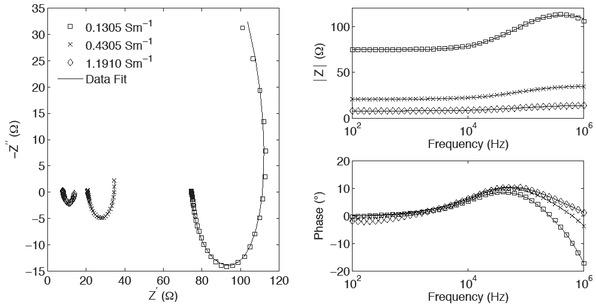Fitting is performed on the impedance spectra of three known conductivity solutions. Artefact consistent with the existence of a parallel conductance is seen in each spectrum and is more pronounced for the lower conductivity solutions.

A good fit between the model and the data is achieved for each solution. The fitted solution resistance (values given in table 2) increases linearly with resistivity. Regression analysis through the origin gives a value of 15.7m-1 for the pseudo geometric factor of the rig.

Finite Element Modelling

Figure 8 shows the sensitivity map of the EndOhm device. The sensitivity is highly non-linear, with distinct positive and negative regions. The sensitivity is large and positive between the upper and lower PU electrodes. In the insulated regions between the PU and CC electrodes are two regions of large negative sensitivity. Away from these dominant regions, the sensitivity goes to zero.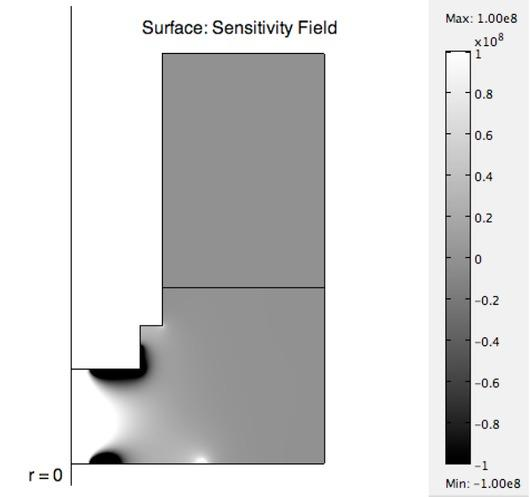The sensitivity map for the EndOhm device shows considerable spatial variation. The sensitivity is large and positive between the PU electrodes. The sensitivity is large and negative between the PU and CC electrodes. Elsewhere the sensitivity tends to zero.

Since this model is axially symmetric, the pseudo geometric factor (or cell constant) can be calculated for the device by integrating over the full 3D volume. The sensitivity based pseudo geometric factor for the device is 13.3m-1. The numerical implementation of the measuring procedure gives a higher pseudo geometric factor of 16.0m-1.

The values estimated for the solution resistance, RS, by fitting to the equivalent circuit model of parallel conductance are shown in table 2 along with the finite element modelling results of expected transfer resistance. There is good agreement between the fitted solution resistance and the numerical estimate, evidenced by the fact that the geometric factors calculated by these two approaches are within 2% of each other. The sensitivity based measurement gives a lower estimate for the geometric factor.

The transfer resistance as calculated using the sensitivity, RSEN; numerical implementation of the measuring procedure, RFEM; through fitting the acquired data to the EEC model, RS. The final row shows the pseudo geometric factor calculated for each approach through linear regression.

Conductivity (S/m)RSEN (Ω)RFEM (Ω)RS (Ω)
0.1305101.90122.80119.72
0.461728.8034.7036.10
1.191011.1713.4514.51
Geometric factor13.3m-116.0m-115.7m-1
Cell Layer Measurements

The optical density measurements confirmed that the number of cells grown on the transwell membranes was proportional to the seeding level used. Micrographs of three transwells depicting different levels of cell growth are shown in figure 9a In the impedance spectra acquired from the transwells, the impedance magnitude is increased with respect to the blank membrane for all transwells on which cells have been cultured. However, the characteristic !dispersion expected for such measurements is not present and their ordering does not always reflect the seeding levels used. Figure 9b shows sample spectra corresonding to the micrographs in the same figure along with the spectra fitted to the equivalent electrical circuit model of parallel conductance. The corresponding reconstructed spectra depicting the impedance from within the measurement rig are shown in figure 9c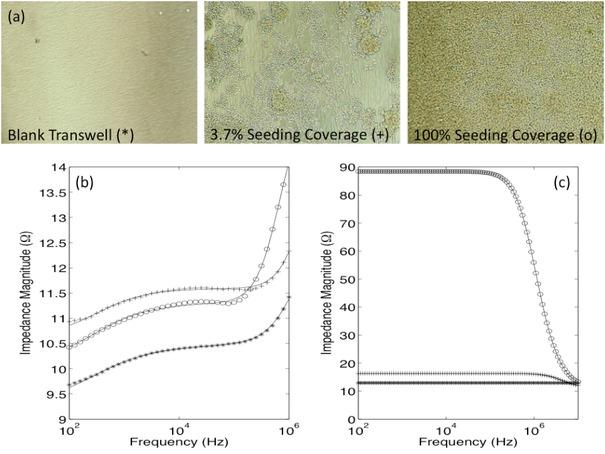Micrographs of three transwell membranes show differences in the final patterns of cell coverage (a). Acquired impedance spectra for these transwells are shown in (b). The solid lines depict the result of fitting this data to the cellular model with parallel conductance. The extracted impedance spectra from within the rig are shown in (c). The characteristic !-dispersion of the cells becomes visible and is more pronounced for the most densely seeded transwell (-o-).

The blank transwell shows no dispersion with frequency meaning that the impedance of the rig is purely resistive, as expected. Dispersions are seen for the other transwells. The magnitude of the dispersion is seen to increase with increasing seeding level. Figure 10 quantifies the dispersion as the drop in impedance from the low frequency limit, i.e. no intracellular flow, to the high frequency limit, i.e. no reactance due to the cell membranes. This drop in impedance is expressed as a percentage of the low frequency limit. No change corresponds to no dispersion and therefore no cellular component contributing whereas a decrease denotes a dispersion and therefore the presence of a cellular comonent. At low cell levels the gaps on the transwell membrane on which no cells have grown dominate. As the confluency of the cell layer increases, the cellular components of the model become more dominant and the magnitude of dispersion increases. The magnitude of the dispersion reaches a maximum as the transwell membrane becomes completely covered by the cell layer.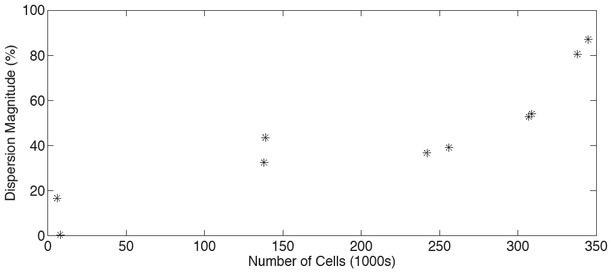The magnitude of the dispersion from the low frequency limit to the high frequency limit rises as the number of cells present on the transwell membrane increases.
Discussion

When making any impedance measurements, it is important to bear in mind that there may be error in the data that does not manifest itself clearly and identifiably. The simulations presented here show that a parallel conductive pathway present in the measurement setup can lead to an underestimation of the sample impedance and may also be characterised by positive phase and an increase in impedance magnitude with frequency, even for purely resistive samples. For the simulated cases classified as having low parallel resistance, i.e. with the resistance of the pathway being equal to that of the sample, the error in the measured resistance was over 50% regardless of the magnitude of the contact impedance. For cases where the electrode impedance is low, the simulations show that there may be no significant dispersion characteristics across the spectrum and yet if a parallel conductive pathway exists, the measured impedance may not be representative of the true impedance of the sample. For example, figure 5 and table 1 show that even in the best case scenario, where the resistance of the parallel pathway is an order of magnitude greater than that of the sample and the polarisation impedance of the CC electrodes is low, there may be little in the data to indicate the artefact (variability less than 2%) while the error in the measured impedance may be as high as 10%. This is a significant error when characterising a sample, and particularly when aiming to detect differences between samples, e.g. healthy versus cancerous tissue.

To minimise the risk of artefact due to a parallel conductive pathway distorting the measured impedance spectrum, the impedance of the CC electrodes should be minimised and the resistance of any parallel pathways maximised.

There are a number of possible reasons for measuring impedance spectra with positive phase and increasing impedance magnitude with frequency, e.g. genuine inductive properties, common mode signals and bridging impedance between the CC and PU electrodes. Such coupling between the electrodes would allow a current to flow directly through the bridging impedance in a manner similar to that described here and can give rise to very large errors even with capacitances as small as 1pF. These errors can also manifest as positive phase, particularly at higher frequencies.

Here we have explored the possibility of using a model of parallel conductance to improve the estimation of the sample's electrical properties. For all of the experimental data shown, a good fit was achieved between the acquired impedance spectra and the model of parallel conductance. Of course, as the number of free parameters increases, so does the risk of fitting to an incorrect model. However, further evidence that parallel conductance may be the source of the artefact is the close agreement between the fitting results and the results of the finite element modelling. This comparison showed the pseudo geometric factor, or cell constant, for the EndOhm device to be within 2% of the numerical value. The sensitivity-based approach appears to under-estimate the pseudo geometric factor and consequently the solution resistance. This may be because of the extreme geometry of the measurement rig in which regions of very high sensitivity gradients, which may not be resolved by the FEM with sufficient accuracy, surround the PU electrodes. The FEM approach is limited if the true electrical properties are unknown and are in fact the focus of the investigation. In such situations, calibration to assess the measurement set-up is vital and known conductivity solutions can be very useful to define regions of operation (15).

The equivalent electrical circuit model was also applied to the spectra obtained from cell cultures grown on transwell membranes. In this case the electrical properties of the samples are unknown. The measured spectra did not correlate with the optical density measurements of cell numbers. However, using the parallel conductance model to improve the estimation of the cell layers' electrical properties allowed information consistent with the cell number measurements to be extracted from the spectra. The magnitude of the dispersion in the impedance spectra reconstructed from the fitting results was quantified and shown to increase as the cell density increased and the gaps on the transwell membranes correspondingly decreased. This is to be expected since the cell membranes act as a barrier to current flow at low frequency but not at high frequency and will therefore introduce a significant frequency dependence that becomes more pronounced as the cell coverage increases and as the purely resistive component of the transwell membrane gaps no longer dominate the impedance of the well chamber.

A more desirable approach to dealing with parallel conductance than modelling would be to remove the error at source. However, in this instance the origin of a parallel conductive pathway is unclear. It may be a fault within the measurement rig; a manifestation of leakage current caused by imperfect matching between the analyser and the measurement rig or by coupling between the electrodes, since many of the features of the artefact presented here are consistent with such a scenario.

Conclusions

The existence of a parallel conductive pathway can be a source of error for bioimpedance measurements. The artefact is characterised by an underestimation of the sample impedance and by a rise in impedance magnitude with frequency concomitant with positive phase. It is most pronounced when the electrode polarisation is high and leads to maximum error when the parallel path is of low resistance, i.e. as it tends toward a shorting of the measurement setup.

In situations where spectra are distorted by artefact consistent with these characteristics, we have shown that equivalent electrical circuit fitting can be used as a means of improving the estimate of the true sample impedance.

#### The transfer resistance as calculated using the sensitivity, RSEN; numerical implementation of the measuring procedure, RFEM; through fitting the acquired data to the EEC model, RS. The final row shows the pseudo geometric factor calculated for each approach through linear regression.

Conductivity (S/m)RSEN (Ω)RFEM (Ω)RS (Ω)
0.1305101.90122.80119.72
0.461728.8034.7036.10
1.191011.1713.4514.51
Geometric factor13.3m-116.0m-115.7m-1

#### The variability of the impedance magnitude is calculated with the range expressed as a percentage of the mean. The mean error of the magnitude is with respect to the true sample impedance.

CaseVariability (%)Mean Error (%)
High ZCC High RP17.0020.35
High ZCC Low RP80.5371.14
Low ZCC High RP1.809.98
Low ZCC Low RP9.5752.53

Grimnes S, Martinsen ØG. Bioimpedance and Bioelectricity Basics. San Diego, CA: Academic Press; 2000.GrimnesSMartinsenØGBioimpedance and Bioelectricity BasicsSan Diego, CAAcademic Press200010.1016/B978-012303260-7/50009-5Search in Google Scholar

Schwan H. Electrode Polarization Impedance and Measurements in biological materials. Annals of the New York Academy of Sciences. 1968 ;148(1):191. Available from: http://www.ncbi.nlm.nih.gov/pubmed/523764110.1111/j.1749-6632.1968.tb20349.x5237641SchwanHElectrode Polarization Impedance and Measurements in biological materialsAnnals of the New York Academy of Sciences19681481191Available fromhttp://www.ncbi.nlm.nih.gov/pubmed/52376415237641

Mazzeo B a. Parasitic capacitance influence of potentialsensing electrodes on four-electrode liquid impedance measurements. Journal of Applied Physics. 2009 ;105(9):094106. Available from: http://link.aip.org/link/JAPIAU/v105/i9/p094106/s110.1063/1.3124365MazzeoB aParasitic capacitance influence of potentialsensing electrodes on four-electrode liquid impedance measurementsJournal of Applied Physics20091059094106Available fromhttp://link.aip.org/link/JAPIAU/v105/i9/p094106/s1

Mazzeo B a, Flewitt AJ. Two- and four-electrode, wide-bandwidth, dielectric spectrometer for conductive liquids: Theory, limitations, and experiment. Journal of Applied Physics. 2007 ;102(10):104106. Available from: http://link.aip.org/link/JAPIAU/v102/i10/p104106/s110.1063/1.2815666MazzeoB aFlewittAJTwo- and four-electrode, wide-bandwidth, dielectric spectrometer for conductive liquids: Theory, limitations, and experimentJournal of Applied Physics200710210104106Available fromhttp://link.aip.org/link/JAPIAU/v102/i10/p104106/s1

Grimnes S, Martinsen ØG. Sources of error in tetrapolar impedance measurements on biomaterials and other ionic conductors. Journal of Physics D: Applied Physics. 2007 Jan ;40(1):9-14. Available from: http://dx.doi.org/10.1088/0022-3727/40/1/S0210.1088/0022-3727/40/1/S02GrimnesSMartinsenØGSources of error in tetrapolar impedance measurements on biomaterials and other ionic conductorsJournal of Physics D: Applied Physics2007401914Available fromhttp://dx.doi.org/10.1088/0022-3727/40/1/S02

Gersing E, Schafer M, Osypka M. The appearance of positive phase angles in impedance measurements on extended biological objects. In: Innovation et technologie en biologie et médecine. ASTEC/ITBM; 1995, p. 71-76. Available from: http://cat.inist.fr/?aModele=afficheN&cpsidt=3694830GersingESchaferMOsypkaMThe appearance of positive phase angles in impedance measurements on extended biological objectsInnovation et technologie en biologie et médecine. ASTEC/ITBM19957176Available fromhttp://cat.inist.fr/?aModele=afficheN&cpsidt=3694830Search in Google Scholar

Geselowitz DB. An application of electrocardiographic lead theory to impedance plethysmography. [Internet]. IEEE transactions on bio-medical engineering. 1971 Jan ;18(1):38-41. Available from: http://www.ncbi.nlm.nih.gov/pubmed/5545046GeselowitzDBAn application of electrocardiographic lead theory to impedance plethysmography. [Internet]IEEE transactions on bio-medical engineering19711813841Available fromhttp://www.ncbi.nlm.nih.gov/pubmed/554504610.1109/TBME.1971.4502787Search in Google Scholar

Brown BH, Wilson AJ, Bertemes-Filho P. Bipolar and tetrapolar transfer impedance measurements from volume conductor. Electronics Letters. 2000 ;36(25):2060. Available from: http://link.aip.org/link/ELLEAK/v36/i25/p2060/s110.1049/el:20001439BrownBHWilsonAJBertemes-FilhoPBipolar and tetrapolar transfer impedance measurements from volume conductorElectronics Letters200036252060Available fromhttp://link.aip.org/link/ELLEAK/v36/i25/p2060/s1

Bronzino JD. The biomedical engineering handbook. 2000. Available from: http://books.google.com/books?id=ijhP6BZxCMACBronzinoJDThe biomedical engineering handbook2000Available fromhttp://books.google.com/books?id=ijhP6BZxCMACSearch in Google Scholar

Wegener J, Keese CR, Giaever I. Electric cell-substrate impedance sensing (ECIS) as a noninvasive means to monitor the kinetics of cell spreading to artificial surfaces. [Internet]. Experimental cell research. 2000 Aug ;259(1):158-66. Available from: http://www.ncbi.nlm.nih.gov/pubmed/1094258810.1006/excr.2000.4919WegenerJKeeseCRGiaeverIElectric cell-substrate impedance sensing (ECIS) as a noninvasive means to monitor the kinetics of cell spreading to artificial surfaces[Internet]. Experimental cell research2000259115866Available fromhttp://www.ncbi.nlm.nih.gov/pubmed/1094258810942588

Brag—s R, Sarro E, Fontova a, Soley a, Cairό J, Bayés-Genίs a, et al. Four versus two-electrode measurement strategies for cell growing and differentiation monitoring using electrical impedance spectroscopy. Conference proceedings: Annual International Conference of the IEEE Engineering in Medicine and Biology Society. IEEE Engineering in Medicine and Biology Society. Conference. 2006 Jan ;12106-9. Available from: http://www.ncbi.nlm.nih.gov/pubmed/17946497Brag—sRSarroEFontovaaSoleyaCairόJBayés-GenίsaFour versus two-electrode measurement strategies for cell growing and differentiation monitoring using electrical impedance spectroscopyConference proceedings: Annual International Conference of the IEEE Engineering in Medicine and Biology SocietyIEEE Engineering in Medicine and Biology Society. Conference2006Jan ;12106-9. Available fromhttp://www.ncbi.nlm.nih.gov/pubmed/1794649710.1109/IEMBS.2006.26028717946497Search in Google Scholar

Lagarias JC, Reeds J a, Wright MH, Wright PE. Convergence Properties of the Nelder--Mead Simplex Method in Low Dimensions. SIAM Journal on Optimization. 1998 ;9(1):112. Available from: http://link.aip.org/link/SJOPE8/v9/i1/p112/s110.1137/S1052623496303470LagariasJCReeds JaWrightMHWrightPEConvergence Properties of the Nelder--Mead Simplex Method in Low DimensionsSIAM Journal on Optimization199891112Available fromhttp://link.aip.org/link/SJOPE8/v9/i1/p112/s1

Fricke H. The theory of electrolytic polarization. Philosophical Magazine Series 7. 1932 ;14(90):310-318. Available from: http://www.informaworld.com/10.1080/1478644320946206410.1080/14786443209462064FrickeHThe theory of electrolytic polarizationPhilosophical Magazine Series 719321490310318Available fromhttp://www.informaworld.com/10.1080/14786443209462064

Brug G, Den Eeden ALG van, Sluyters-Rehbach M, Sluyters J. The analysis of electrode impedances complicated by the presence of a constant phase element. Journal of Electroanalytical Chemistry. 1984 ;176(1-2):275-295. Available from: http://dx.doi.org/10.1016/S0022-0728(84)80324-110.1016/S0022-0728(84)80324-1BrugGDenEeden ALG vanSluyters-RehbachMSluytersJThe analysis of electrode impedances complicated by the presence of a constant phase elementJournal of Electroanalytical Chemistry19841761-2275295Available fromhttp://dx.doi.org/10.1016/S0022-0728(84)80324-1

Pliquett U, Frense D, Schönfeldt M, Fratzer C, Zhang Y, Cahill B, et al. Testing miniaturized electrodes for impedance measurements within the beta-dispersion - a practical approach. Journal of Electrical Bioimpedance. 2010 ;141-55. Available from: http://tinyurl.com/2vuaea2PliquettUFrenseDSchönfeldtMFratzerCZhangYCahillBTesting miniaturized electrodes for impedance measurements within the beta-dispersion - a practical approachJournal of Electrical Bioimpedance201014155Available fromhttp://tinyurl.com/2vuaea2Search in Google Scholar

Articles recommandés par Trend MD